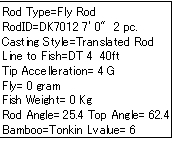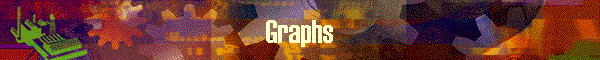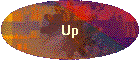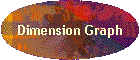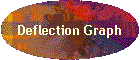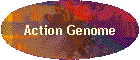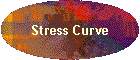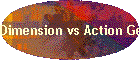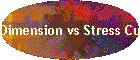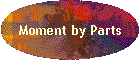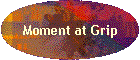This panel shows graphic output which are listed in the navigation at left.

The calculation results and graphs are all recorded in the output excel file.

Output Excel file is stored in the "Data" Folder of Dynarod with the name of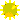Rod ID.xls

### Comparison Function

From version 2.92, the rod which is under calculation can compare against another rods which were already calculated one by one.

 Item Meaning Compare with Originally left blank. This field is combo box and shows pull down list. This feature allows you to compare the current rod to any of rod which reside in Archive, and calculation is completed. In the case the specified rod is not generate the excel output of [Deflection / Stress], message will prompt to the effect. As a hidden option, you can compare the current rod with the rod in existing excel output files in data folder.  In this case, specify the name of excel file like, defxxxxxx or strxxxxxx with no file extension. (xxxxxx is rod id). Reset This button clears the compared RodID and the graphs display solely the original rod. Comparison is available for the graphs of Taper, Deflection, Action Genome, and Stress Curve.

### Deflection and Action Genome

DynaRod uses the following measurement to represent the rod deflection.

Rod Angle

As shown in the graph below, rod angle is the angle which is made between vertical line and the line which connect butt end and tip top.

The less this angle is, the stiffer (or faster) the rod becomes.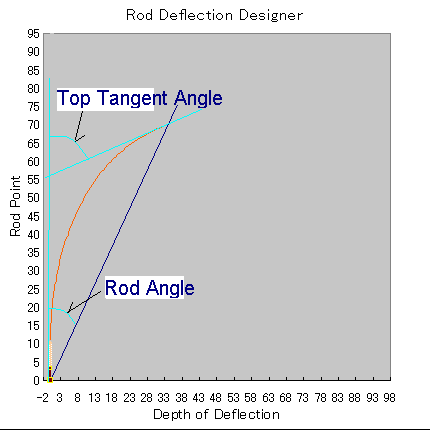Rod Stiffness

The existing definition of rod action type, such as "Fast action is the rod whose 30 % of tip section deflects", may not be applied for any parabolic action rod.  It would be necessary to implement the standardized measurement for any kind of action rod.

DynaRod proposes to implement a new measure of rod stiffness based on the Rod Angle as follows;

"The Rod Stiffness is defined by Rod Angle which is calculated or measured under an standardized load."rod is under the load of 4G accelerationrod is moved translatedif calculated result is used, it should be by dynamic calculationLine to Fish is assumed as follows;#3    30 feet#4    40 feet#5    50 feet#6    60 feet#7    70 feet#8    80 feet#9    90 feet
 Rod Angle Rod Stiffness -15° very Hard 15°-20° Hard 20°-25° Medium Hard 25°-30° Medium 30°-35° Medium Soft 35°-40° Soft 40°- Very Soft

This is to describe a rod stiffness, not rod action.  For rod action, Action Genome is measure for the proposed type of action.

### Tip Top Tangent Angle

The Angle made between a vertical line and tangent of the 1st 1" of the tip top when an entire rod bend is measured in 1".

According to the physical rule, this angle represent the total of each bend angle for each 1" section of the rod.  The 1" section's bend angle is measured against the angle of 1" prior section.  So Top Tangent Angle can be said as the total of rod bends or magnitude of bend angle.

### Action Genome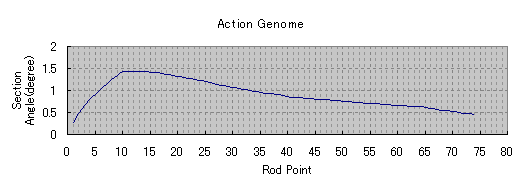The Angle made between a vertical line and tangent of the 1st 1" of the tip top when an entire rod bend is measured in 1".

According to the physical rule, this angle represent the total of each bend angle for each 1" section of the rod.  The 1" section's bend angle is measured against the angle of 1" prior section.  So Top Tangent Angle can be said as the total of rod bends or magnitude of bend angle.

This action genome can be understood that it shows the exact bend angle from previous 1" section.  The higher part of the graph bends more.

The benefit of those graphs as the design tool, arewe can see both graphs at the same time and co-relatedwe can reverse calculate the dimension from the action genome

Deflection Designer tool is good to clarify the fly fishers requirements for a rod.

### Assumptions of calculation

Each graph carries the assumed information when it is calculated at the upper left corner as shown below.

This is to compare with the current information in the Archive if some data is changed or not.

The following data items are printed;

Rod Type, Rod ID, Rod Length, no of pieces, assumed Casting Style, Line Type, Line Wt, Line to Fish, Tip acceleration in G, weight of Fly, weight of Fish, Rod Angle, Top Angle, used Bamboo, used Lvalue.  Note: Top Angle is for tip top tangent angle.